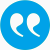#Löhne, Andreas, Rudloff, Birgit. 2014. An algorithm for calculating the set of superhedging portfolios in markets with transaction costs. Int. J. Theor. Appl. Finan. 17 (02): S. 1450012-[33 p].

BibTeX

## Abstract

We study the explicit calculation of the set of superhedging portfolios of contingent claims in a discrete-time market model for d assets with proportional transaction costs. The set of superhedging portfolios can be obtained by a recursive construction involving set operations, going backward in the event tree. We reformulate the problem as a sequence of linear vector optimization problems and solve it by adapting known algorithms. The corresponding superhedging strategy can be obtained going forward in the tree. Examples are given involving multiple correlated assets and basket options. Furthermore, we relate existing algorithms for the calculation of the scalar superhedging price to the set-valued algorithm by a recent duality theory for vector optimization problems. The main contribution of the paper is to establish the connection to linear vector optimization, which allows to solve numerically multi-asset superhedging problems under transaction costs.

## Tags

Press 'enter' for creating the tag

## Publication's profile

Status of publication Published WU Journal article International Journal of Theoretical and Applied Finance A FIN-A, STRAT-B, VW-D, WH-B English An algorithm for calculating the set of superhedging portfolios in markets with transaction costs 17 02 2014 1450012 [33 p] Y http://arxiv.org/pdf/1107.5720 http://dx.doi.org/10.1142/S0219024914500125

## Associations

People
Rudloff, Birgit (Details)
External
Löhne, Andreas (Friedrich-Schiller-Universität Jena, Germany)